SBAS404D October   2006  – February 2016

PRODUCTION DATA.

1. Features
2. Applications
3. Description
4. Revision History
5. Pin Configuration and Functions
6. Specifications
7. Detailed Description
1. 7.1 Overview
2. 7.2 Functional Block Diagram
3. 7.3 Feature Description
1. 7.3.1 Analog
2. 7.3.2 Digital
4. 7.4 Device Functional Modes
5. 7.5 Register Maps
8. Application and Implementation
1. 8.1 Application Information
2. 8.2 Typical Application
9. Power Supply Recommendations
10. 10Layout
11. 11Device and Documentation Support
12. 12Mechanical, Packaging, and Orderable Information

• PM|64

## 6 Specifications

### 6.1 Absolute Maximum Ratings

over operating free-air temperature range (unless otherwise noted)(1)
MIN MAX UNIT
Supply voltage, HVDD to AGND –0.3 18 V
Supply voltage, HVSS to AGND –18 0.3 V
Supply voltage, AVDD to AGND –0.3 6 V
Supply voltage, BVDD to BGND –0.3 6 V
Reference input voltage with respect to AGND AGND – 0.3 AVDD + 0.3 V
Digital input voltage with respect to BGND BGND – 0.3 BVDD + 0.3 V
Ground voltage difference AGND to BGND ±0.3 V
Input current to all pins except supply –10 10 mA
Maximum virtual junction temperature, TJ 150 °C
Storage Temperature, Tstg -65 150 °C
(1) Stresses beyond those listed under absolute maximum ratings may cause permanent damage to the device. These are stress ratings only and functional operation of the device at these or any other conditions beyond those indicated under recommended operating conditions is not implied. Exposure to absolute-maximum-rated conditions for extended periods may affect device reliability.

### 6.2 ESD Ratings

VALUE UNIT
V(ESD) Electrostatic discharge Human-body model (HBM), per ANSI/ESDA/JEDEC JS-001
JEDEC standard 22, test method A114-C.01(1)
±2000 V
Charged-device model (CDM), per JEDEC specification JESD22-C101
JEDEC standard 22, test method C101(2)
±500
(1) JEDEC document JEP155 states that 500-V HBM allows safe manufacturing with a standard ESD control process.
(2) JEDEC document JEP157 states that 250-V CDM allows safe manufacturing with a standard ESD control process.

### 6.3 Recommended Operating Conditions

MIN NOM MAX UNIT
Supply voltage, AVDD to AGND 4.5 5 5.5 V
Supply voltage, BVDD to BGND Low-voltage levels 2.7 3.0 3.6 V
5-V logic levels 4.5 5 5.5
Input supply voltage, HVDD to AGND Range 1 (±2 × VREF) 2 × VREF 16.5 V
Range 2 (±4 × VREF) 4 × VREF 16.5
Input supply voltage, HVSS to AGND Range 1 (±2 × VREF) –16.5 –2 × VREF V
Range 2 (±4 × VREF) –16.5 –4 × VREF
Reference input voltage (VREF) 0.5 2.5 3.0 V
Analog inputs(1) Range 1 (±2 × VREF) –2 × VREF 2 × VREF V
Range 1 (±4 × VREF) –4 × VREF 4 × VREF
Operating ambient temperature, TA –40 125 °C

### 6.4 Thermal Information

PM (LQFP)
64 PINS
RθJA Junction-to-ambient thermal resistance High-K thermal resistance(2) 50.3 °C/W
RθJC(top) Junction-to-case (top) thermal resistance 12.0 °C/W
RθJB Junction-to-board thermal resistance 24.0 °C/W
ψJT Junction-to-top characterization parameter 0.5 °C/W
ψJB Junction-to-board characterization parameter 23.5 °C/W
RθJC(bot) Junction-to-case (bottom) thermal resistance NA °C/W
(2) Modeled in accordance with the Low-K or High-K thermal metric definitions of EIA/JESD51-3.

### 6.5 Electrical Characteristics: General

over recommended operating free-air temperature range of –40°C to 125°C, AVDD = 4.5 V to 5.5 V, BVDD = 2.7 V to 5.5 V, HVDD = 10 V to 15 V, HVSS = –15 V to –10 V, VREF = 2.5 V (internal), and fDATA = maximum (unless otherwise noted)
PARAMETER TEST CONDITIONS MIN TYP(1) MAX UNIT
CHXX Bipolar full-scale range RANGE pin/RANGE bit = 0 –4 × VREF 4 × VREF V
RANGE pin/RANGE bit = 1 –2 × VREF 2 × VREF
Input capacitance Input range = ±4 × VREF 10 pF
Input range = ±2 × VREF 20
Input leakage current No ongoing conversion ±1 μA
Aperture delay 5 ns
Aperture delay matching Common CONVST for all channels 250 ps
Aperture jitter 50 ps
EXTERNAL CLOCK INPUT (XCLK)
fXCLK External clock frequency An external reference must be used for fXCLK > fCCLK 1 18 20 MHz
External clock duty cycle 45% 55%
REFERENCE VOLTAGE OUTPUT (REFOUT)
VREF Reference voltage 2.5-V operation, REFDAC = 0x3FF 2.485 2.5 2.515 V
2.5-V operation, REFDAC = 0x3FF at 25°C 2.496 2.5 2.504
3-V operation, REFDAC = 0x3FF 2.985 3.0 3.015
3-V operation, REFDAC = 0x3FF at 25°C 2.995 3.0 3.005
dVREF/dT Reference voltage drift ±10 ppm/°C
PSRR Power-supply rejection ratio 73 dB
IREFOUT Output current With dc current –2 2 mA
IREFSC Short-circuit current(2) 50 mA
tREFON Turn-on settling time 10 ms
External load capacitance At CREF_x pins 4.7 10 μF
At REFIO pins 100 470
REFDAC Tuning range Internal reference output voltage range 0.2 × VREF VREF V
REFDAC resolution 10 Bits
DNLDAC REFDAC differential nonlinearity –1 ±0.1 1 LSB
INLDAC REFDAC integral nonlinearity –2 ±0.1 2 LSB
VOSDAC REFDAC offset error VREF = 0.5 V (DAC = 0x0CC) –4 ±0.65 4 LSB
REFERENCE VOLTAGE INPUT (REFIN)
VREFIN Reference input voltage 0.5 2.5 3.025 V
Input resistance 100
Input capacitance 5 pF
Reference input current 1 μA
SERIAL CLOCK INPUT (SCLK)
fSCLK Serial clock input frequency 0.1 36 MHz
tSCLK Serial clock period 0.0278 10 μs
Serial clock duty cycle 40% 60%
DIGITAL INPUTS(3)
Logic family CMOS with Schmitt-Trigger
High-level input voltage 0.7 × BVDD BVDD + 0.3 V
Low-level input voltage BGND – 0.3 0.3 × BVDD V
Input current VI = BVDD to BGND –50 50 nA
Input capacitance 5 pF
DIGITAL OUTPUTS(3)
Logic family CMOS
High-level output voltage IOH = 100 μA BVDD – 0.6 BVDD V
Low-level output voltage IOH = –100 μA BGND BGND + 0.4 V
High-impedance-state output current –50 50 nA
Output capacitance 5 pF
POWER-SUPPLY REQUIREMENTS
AVDD Analog supply voltage 4.5 5 5.5 V
BVDD Buffer I/O supply voltage 2.7 3 5.5 V
HVDD Input positive supply voltage 5 10 16.5 V
HVSS Input negative supply voltage –16.5 –10 –5 V
IAVDD Analog supply current(4) fDATA = maximum 30 36 mA
ADS8556, fDATA = 250 kSPS (auto-NAP mode) 14 16.5
ADS8557, fDATA = 250 kSPS (auto-NAP mode) 14 17
ADS8558, fDATA = 250 kSPS (auto-NAP mode) 14 18
Auto-NAP mode, no ongoing conversion, internal conversion clock 4 6
Power-down mode 0.1 50 μA
IBVDD Buffer I/O supply current(5) fDATA = maximum 0.9 2 mA
fDATA = 250 kSPS (auto-NAP mode) 0.5 1.5
Auto-NAP mode, no ongoing conversion, internal conversion clock 0.1 10 μA
Power-down mode 0.1 10
IHVDD Input positive supply current(6) ADS8556, fDATA = maximum 3 3.5 mA
ADS8557, fDATA = maximum 3.1 3.6
ADS8558, fDATA = maximum 3.3 4
fDATA = 250 kSPS (auto-NAP mode) 1.6 2
Auto-NAP mode, no ongoing conversion, internal conversion clock 0.2 0.3 μA
Power-down mode 0.1 10
IHVSS Input negative supply current(7) ADS8556, fDATA = maximum 3.6 4 mA
ADS8557, fDATA = maximum 3.6 4.2
ADS8558, fDATA = maximum 4 4.8
fDATA = 250 kSPS (auto-NAP mode) 1.8 2.2
Auto-NAP mode, no ongoing conversion, internal conversion clock 0.2 0.25 μA
Power-down mode 0.1 10
Power dissipation(8) ADS8556, fDATA = maximum 251.7 298.5 mW
ADS8557, fDATA = maximum 253.2 303
ADS8558, fDATA = maximum 262.2 318
ADS8556, fDATA = 250 kSPS (auto-NAP mode) 122.5 150
ADS8557, fDATA = 250 kSPS (auto-NAP mode) 122.5 152.5
ADS8558, fDATA = 250 kSPS (auto-NAP mode) 122.5 157.5
Auto-NAP mode, no ongoing conversion, internal conversion clock 26 38.3
Power-down mode 3.8 580 μW
(1) All values are at TA = 25°C.
(2) Reference output current is not limited internally.
(3) Specified by design.
(4) At AVDD = 5 V.
(5) At BVDD = 3 V, parallel mode, load capacitance = 6 pF per pin.
(6) At HVDD = 15 V.
(7) At HVSS = –15 V.
(8) At AVDD = 5 V, BVDD = 3 V, HVDD = 15 V, and HVSS = –15 V.

over recommended operating free-air temperature range of –40°C to 125°C, AVDD = 4.5 V to 5 V, BVDD = 2.7 V to 5.5 V, HVDD = 10 V to 15 V, HVSS = –15 V to –10 V, VREF = 2.5 V (internal), and fDATA = 630 kSPS in parallel mode or 450 kSPS in serial mode (unless otherwise noted)
PARAMETER TEST CONDITIONS MIN TYP(1) MAX UNIT
DC ACCURACY
Resolution 16 Bits
No missing codes 16 Bits
INL Integral linearity error At TA = –40°C to 85°C –3 ±1.5 3 LSB
At TA = –40°C to 125°C –4 ±1.5 4
DNL Differential linearity error At TA = –40°C to 85°C –1 ±0.75 1.5 LSB
At TA = –40°C to 125°C –1 ±0.75 2
Offset error –4 ±0.8 4 mV
Offset error drift ±3.5 μV/°C
Gain error Referenced to voltage at REFIO –0.75 ±0.25 0.75 %FSR
Gain error drift Referenced to voltage at REFIO ±6 ppm/°C
PSRR Power-supply rejection ratio At output code FFFFh, related to AVDD 60 dB
SAMPLING DYNAMICS
tACQ Acquisition time 280 ns
tCONV Conversion time per ADC 1.26 μs
tCCLK Internal conversion clock period 18.5 tCCLK
68.0 ns
fDATA Throughput rate Parallel interface, internal clock and reference 630 kSPS
Serial interface, internal clock and reference 450
AC ACCURACY
SNR Signal-to-noise ratio At fIN = 10 kHz, TA = –40°C to 85°C 90 91.5 dB
At fIN = 10 kHz, TA = –40°C to 125°C 89 91.5
SINAD Signal-to-noise ratio + distortion At fIN = 10 kHz, TA = –40°C to 85°C 87 89.5 dB
At fIN = 10 kHz, TA = –40°C to 125°C 86.5 89.5
THD Total harmonic distortion(2) At fIN = 10 kHz, TA = –40°C to 85°C –94 –90 dB
At fIN = 10 kHz, TA = –40°C to 125°C –94 –89.5
SFDR Spurious-free dynamic range At fIN = 10 kHz, TA = –40°C to 85°C 90 95 dB
At fIN = 10 kHz, TA = –40°C to 125°C 89.5 95
Channel-to-channel isolation At fIN = 10 kHz 100 dB
–3-dB small-signal bandwidth In 4 × VREF mode 48 MHz
In 2 × VREF mode 24
(1) All values are at TA = 25°C.
(2) Calculated on the first nine harmonics of the input frequency.

over recommended operating free-air temperature range of –40°C to 125°C, AVDD = 4.5 V to 5.5 V, BVDD = 2.7 V to 5.5 V, HVDD = 10 V to 15 V, HVSS = –15 V to –10 V, VREF = 2.5 V (internal), and fDATA = 670 kSPS in parallel mode or 470 kSPS in serial mode (unless otherwise noted)
PARAMETER TEST CONDITIONS MIN TYP(1) MAX UNIT
DC ACCURACY
Resolution 14 Bits
No missing codes 14 Bits
INL Integral linearity error –1 ±0.4 1 LSB
DNL Differential linearity error –1 ±0.25 1 LSB
Offset error –4 ±0.8 4 mV
Offset error drift ±3.5 μV/°C
Gain error Referenced to voltage at REFIO –0.75 ±0.25 0.75 %FSR
Gain error drift Referenced to voltage at REFIO ±6 ppm/°C
PSRR Power-supply rejection ratio At output code FFFFh, related to AVDD 60 dB
SAMPLING DYNAMICS
tACQ Acquisition time 280 ns
tCONV Conversion time per ADC 1.19 μs
tCCLK Internal conversion clock period 18.5 tCCLK
64.1 ns
fDATA Throughput rate Parallel interface, internal clock and reference 670 kSPS
Serial interface, internal clock and reference 470
AC ACCURACY
SNR Signal-to-noise ratio At fIN = 10 kHz 84 85 dB
SINAD Signal-to-noise ratio + distortion At fIN = 10 kHz 83 84 dB
THD Total harmonic distortion(2) At fIN = 10 kHz –91 –86 dB
SFDR Spurious-free dynamic range At fIN = 10 kHz 86 92 dB
Channel-to-channel isolation At fIN = 10 kHz 100 dB
–3-dB small-signal bandwidth In 4 × VREF mode 48 MHz
In 2 × VREF mode 24
(1) All values are at TA = 25°C.
(2) Calculated on the first nine harmonics of the input frequency.

over recommended operating free-air temperature range of –40°C to 125°C, AVDD = 4.5 V to 5 V, BVDD = 2.7 V to 5.5 V, HVDD = 10 V to 15 V, HVSS = –15 V to –10 V, VREF = 2.5 V (internal), and fDATA = 730 kSPS in parallel mode or 500 kSPS in serial mode (unless otherwise noted)
PARAMETER TEST CONDITIONS MIN TYP(1) MAX UNIT
DC ACCURACY
Resolution 12 Bits
No missing codes 12 Bits
INL Integral linearity error –0.75 ±0.2 0.75 LSB
DNL Differential linearity error –0.5 ±0.2 0.5 LSB
Offset error –4 ±0.8 4 mV
Offset error drift ±3.5 μV/°C
Gain error Referenced to voltage at REFIO –0.75 ±0.25 0.75 %FSR
Gain error drift Referenced to voltage at REFIO ±6 ppm/°C
PSRR Power-supply rejection ratio At output code FFFFh, related to AVDD 60 dB
SAMPLING DYNAMICS
tACQ Acquisition time 280 ns
tCONV Conversion time per ADC 1.09 μs
tCCLK Internal conversion clock period 18.5 tCCLK
58.8 ns
fDATA Throughput rate Parallel interface, internal clock and reference 730 kSPS
Serial interface, internal clock and reference 500
AC ACCURACY
SNR Signal-to-noise ratio At fIN = 10kHz 73 73.9 dB
SINAD Signal-to-noise ratio + distortion At fIN = 10kHz 73 73.8 dB
THD Total harmonic distortion(2) At fIN = 10kHz –89 –84 dB
SFDR Spurious-free dynamic range At fIN = 10kHz 84 92 dB
Channel-to-channel isolation At fIN = 10kHz 100 dB
–3-dB small-signal bandwidth In 4 × VREF mode 48 MHz
In 2 × VREF mode 24
(1) All values are at TA = 25°C.
(2) Calculated on the first nine harmonics of the input frequency.

### 6.9 Power Dissipation Characteristics

over operating free-air temperature range (unless otherwise noted)
PARAMETER TEST CONDITIONS MIN TYP MAX UNIT
PD Device power dissipation ADS8556, HVDD = 15 V, HVSS = –15 V, AVDD = 5 V, BVDD = 3 V, and fDATA = maximum 251.7 298.5 mW
ADS8557, HVDD = 15 V, HVSS = –15 V, AVDD = 5 V, BVDD = 3 V, and fDATA = maximum 253.2 303.0
ADS8558, HVDD = 15 V, HVSS = –15 V, AVDD = 5 V, BVDD = 3 V, and fDATA = maximum 262.2 318.0

### 6.10 Serial Interface Timing Requirements

over recommended operating free-air temperature range at –40°C to 125°C, AVDD = 5 V, and BVDD = 2.7 V to 5.5 V (unless otherwise noted)(1)
MIN NOM MAX UNIT
tACQ Acquisition time 280 ns
tCONV Conversion time ADS8556 1.26 µs
t1 CONVST_x low time 20 ns
t2 BUSY low to FS low time 0 ns
t3 Bus access finished to next conversion start time ADS8556 40 ns
tD1 CONVST_x high to BUSY high delay 5 20 ns
tD2 FS low to SDO_x active delay 5 12 ns
tD3 SCLK rising edge to new data valid delay 15 ns
tD4 FS high to SDO_x 3-state delay 10 ns
tH1 Input data to SCLK falling edge hold time 5 ns
tH2 Output data to SCLK rising edge hold time 5 ns
tS1 Input data to SCLK falling edge setup time 3 ns
tS3 CONVST_x high to XCLK falling or rising edge setup time 6 ns
tSCLK Serial clock period 0.0278 10 μs
(1) All input signals are specified with tR = tF = 1.5 ns (10% to 90% of BVDD) and timed from a voltage level of (VIL + VIH) / 2.

### 6.11 Parallel Interface Timing Requirements (Read Access)

over recommended operating free-air temperature range at –40°C to 125°C, AVDD = 5 V, and BVDD = 2.7 V to 5.5 V (unless otherwise noted)(1)
MIN NOM MAX UNIT
tACQ Acquisition time 280 ns
tCONV Conversion time ADS8556 1.26 µs
t1 CONVST_x low time 20 ns
t2 BUSY low to CS low time 0 ns
t3 Bus access finished to next conversion start time(2) ADS8556 40 ns
t4 CS low to RD low time 0 ns
t5 RD high to CS high time 0 ns
t6 RD pulse duration 30 ns
t7 Minimum time between two read accesses 10 ns
tD1 CONVST_x high to BUSY high delay 5 20 ns
tD5 RD falling edge to output data valid delay 20 ns
tH3 Output data to RD rising edge hold time 5 ns
(1) All input signals are specified with tR = tF = 1.5 ns (10% to 90% of BVDD) and timed from a voltage level of (VIL + VIH) / 2.
(2) Refer to the CS signal or RD, whichever occurs first.

### 6.12 Parallel Interface Timing Requirements (Write Access)

over recommended operating free-air temperature range at –40°C to 125°C, AVDD = 5 V, and BVDD = 2.7 V to 5.5 V (unless otherwise noted)(1)
MIN NOM MAX UNIT
t8 CS low to WR low time 0 ns
t9 WR low pulse duration 15 ns
t10 WR high pulse duration 10 ns
t11 WR high to CS high time 0 ns
tS2 Output data to WR rising edge setup time 5 ns
tH4 Data output to WR rising edge hold time 5 ns
(1) All input signals are specified with tR = tF = 1.5 ns (10% to 90% of BVDD) and timed from a voltage level of (VIL + VIH) / 2.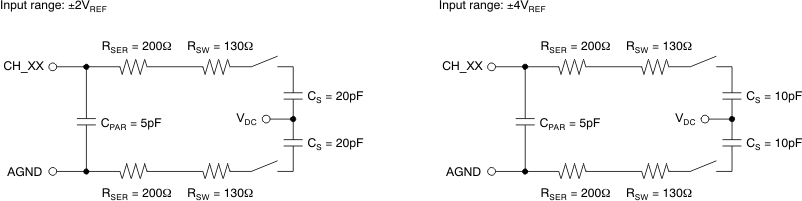Figure 1. Equivalent Input CircuitsFigure 2. Serial Operation Timing Diagram (All Three SDOs Active)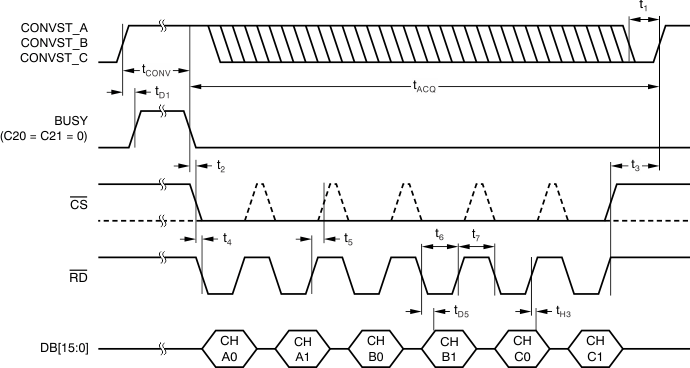Figure 3. Parallel Read Access Timing Diagram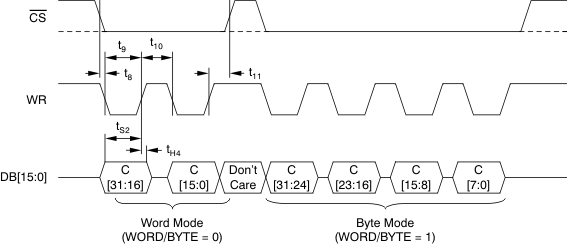Figure 4. Parallel Write Access Timing Diagram

### 6.13 Typical Characteristics

at 25°C, over entire supply voltage range, VREF = 2.5 V (internal), and fDATA = maximum (unless otherwise noted)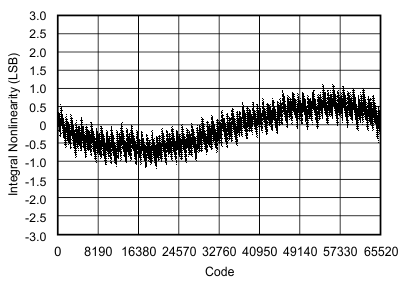AVDD = BVDD = 5 V HVSS = –15 V HVDD = 15 V fDATA = max Internal reference
Figure 5. INL vs Code (ADS8556 ±10-VIN Range)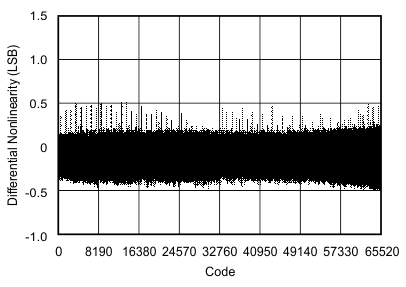AVDD = BVDD = 5 V HVSS = –15 V HVDD = 15 V fDATA = max Internal reference
Figure 7. DNL vs Code (ADS8556 ±10-VIN Range)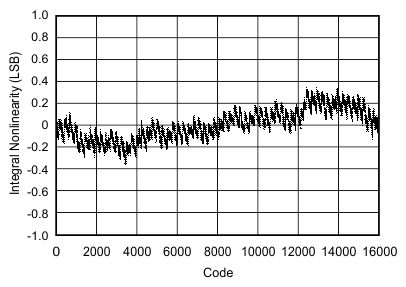AVDD = BVDD = 5 V HVSS = –15 V HVDD = 15 V fDATA = max Internal reference
Figure 9. INL vs Code (ADS8557 ±10-VIN Range)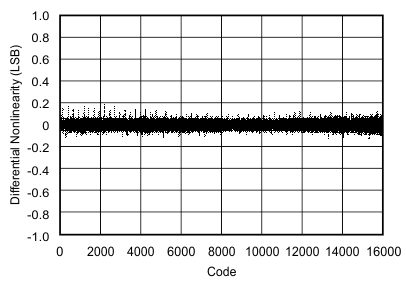AVDD = BVDD = 5 V HVSS = –15 V HVDD = 15 V fDATA = max Internal reference
Figure 11. DNL vs Code (ADS8557 ±10-VIN Range)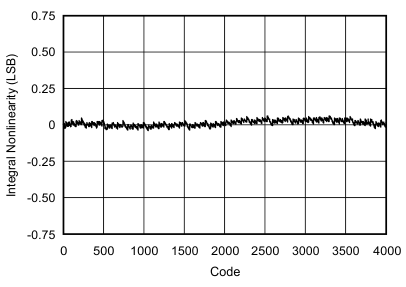AVDD = BVDD = 5 V HVSS = –15 V HVDD = 15 V fDATA = max Internal reference
Figure 13. INL vs Code (ADS8558)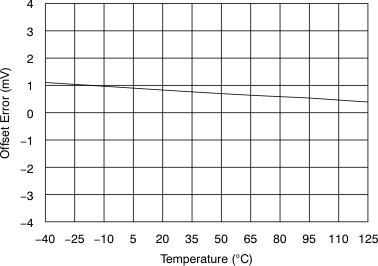Figure 15. Offset Error vs Temperature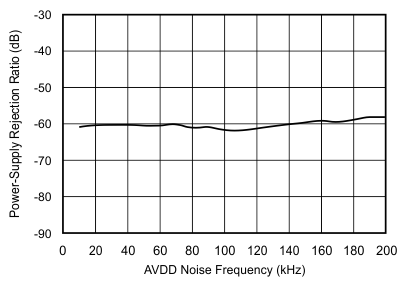CSUPPLY = 100 nF on AVDD
Figure 17. PSRR vs AVDD Noise Frequency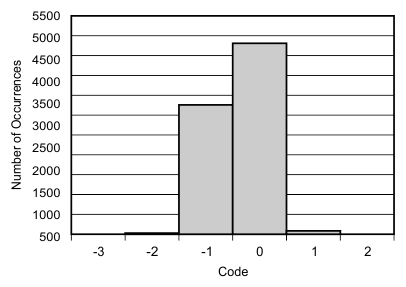AVDD = BVDD = 5 V HVSS = –15 V HVDD = 15 V Range = 4 × VREF 8192 samples Internal reference
Figure 19. Code Histogram (8192 Hits)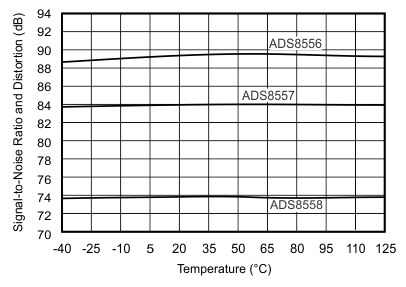AVDD = BVDD = 5 V HVSS = –15 V HVDD = 15 V Range = ±4 × VREF fSIGNAL = 10 kHz fDATA = max Internal reference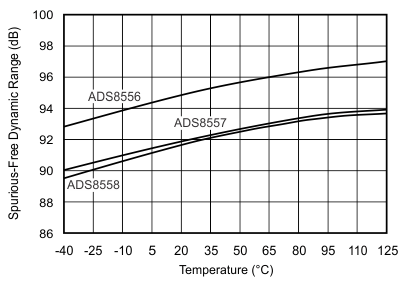AVDD = BVDD = 5 V HVSS = –15 V HVDD = 15 V Range = ±4 × VREF fSIGNAL = 10 kHz fDATA = max Internal reference
Figure 23. SFDR vs Temperature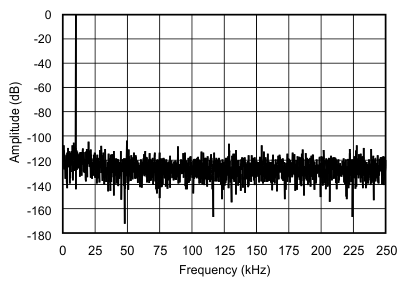AVDD = BVDD = 5 V fSAMPLE = 500 kSPS HVSS = –15 V Range = ±2 × VREF fSIGNAL = 10 kHz HVDD = 15 V Internal reference
Figure 25. Frequency Spectrum
(2048-Point FFT, fIN = 10 kHz, ±5-VIN Range)Figure 27. Internal Reference Voltage vs
Analog Supply Voltage (2.5-V Mode)Figure 29. Internal Reference Voltage vs
Temperature (3-V Mode)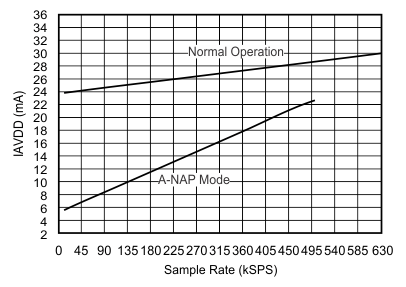AVDD = 5 V Internal reference
Figure 31. ADS8556 Analog Supply Current vs Data Rate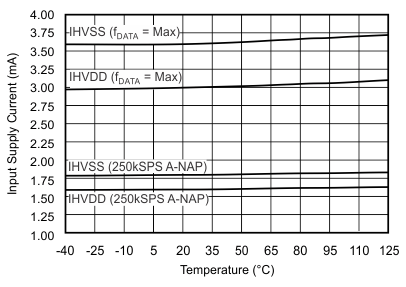Range = ±4 × VREF HVSS = –15 V HVDD = 15 V Internal reference
Figure 33. ADS8556 Input Supply Current vs Temperature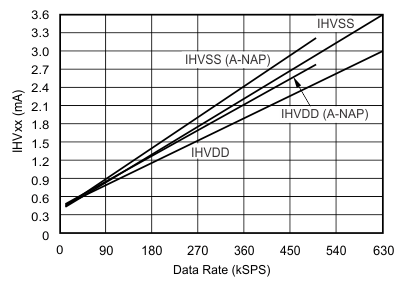Range = ±4 × VREF HVSS = –15 V HVDD = 15 V
Figure 35. ADS8556 Input Supply Current vs Data Rate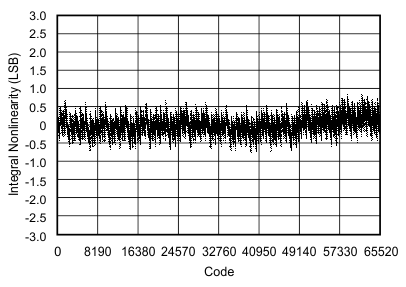AVDD = BVDD = 5 V HVSS = –15 V HVDD = 15 V fDATA = max Internal reference
Figure 6. INL vs Code (ADS8556 ±5-VIN Range)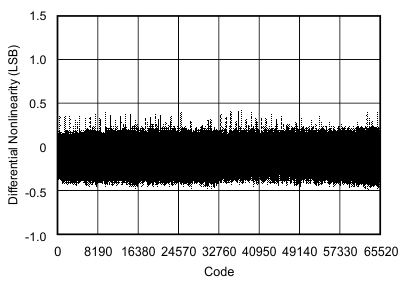AVDD = BVDD = 5 V HVSS = –15 V HVDD = 15 V fDATA = max Internal reference
Figure 8. DNL vs Code (ADS8556 ±5-VIN Range)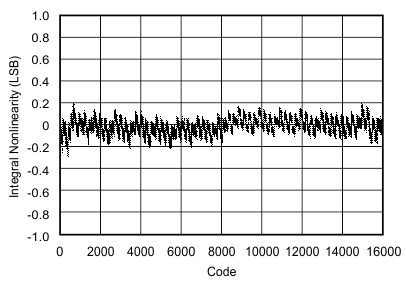AVDD = BVDD = 5 V HVSS = –15 V HVDD = 15 V fDATA = max Internal reference
Figure 10. INL vs Code (ADS8557 ±5-VIN Range)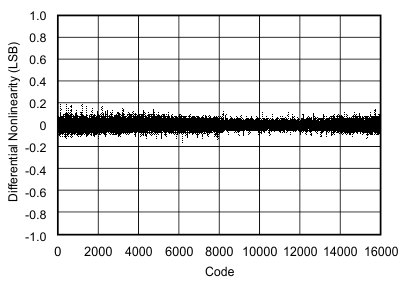AVDD = BVDD = 5 V HVSS = –15 V HVDD = 15 V fDATA = max Internal reference
Figure 12. DNL vs Code (ADS8557 ±5-VIN Range)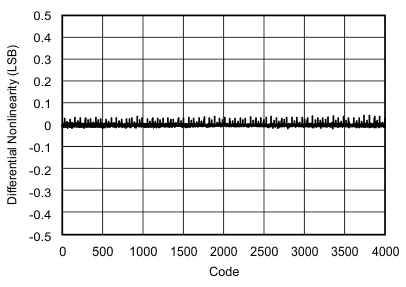AVDD = BVDD = 5 V HVSS = –15 V HVDD = 15 V fDATA = max Internal reference
Figure 14. DNL vs Code (ADS8558)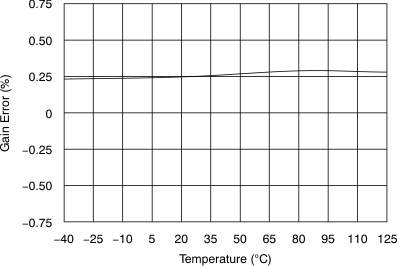Figure 16. Gain Error vs Temperature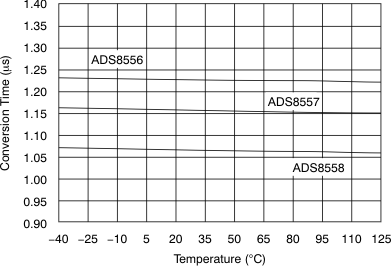Figure 18. Conversion Time vs TemperatureAVDD = BVDD = 5 V HVSS = –15 V HVDD = 15 V Range = ±4 × VREF fSIGNAL = 10 kHz fDATA = max Internal reference
Figure 20. SNR vs Temperature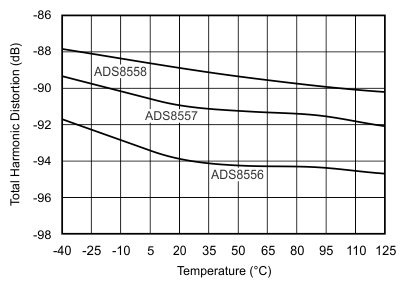AVDD = BVDD = 5 V HVSS = –15 V HVDD = 15 V Range = ±4 × VREF fSIGNAL = 10 kHz fDATA = max Internal reference
Figure 22. THD vs Temperature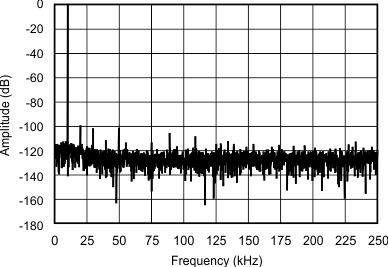AVDD = BVDD = 5 V fSAMPLE = 500 kSPS HVSS = –15 V Range = ±4 × VREF fSIGNAL = 10 kHz HVDD = 15 V Internal reference
Figure 24. Frequency Spectrum
(2048-Point FFT, fIN = 10 kHz, ±10-VIN Range)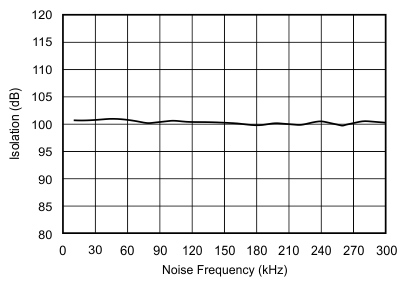AVDD = BVDD = 5 V HVSS = –15 V HVDD = 15 V Range = ±2 × VREF fDATA = max Internal reference
Figure 26. Channel-to-Channel Isolation vs
Input Noise Frequency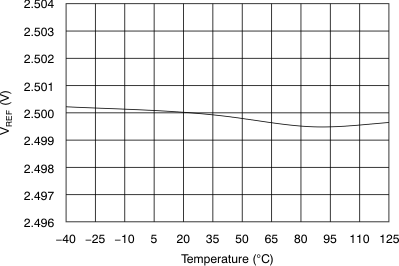Figure 28. Internal Reference Voltage vs
Temperature (2.5-V Mode)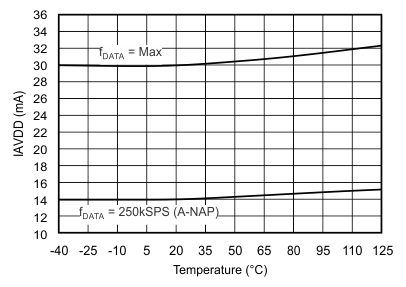AVDD = 5 V Internal reference
Figure 30. Analog Supply Current vs Temperature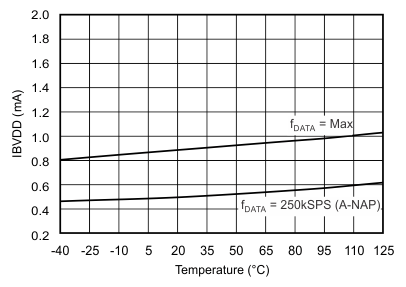BVDD = 5 V
Figure 32. Buffer I/O Supply Current vs Temperature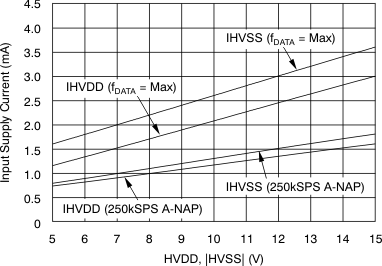Figure 34. ADS8556 Input Supply Current vs
Input Supply Voltage